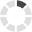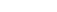### Site InformationLoading... Please wait...

# ECET 299 Week 8 Final Exam

\$24.99

## Product Description

ECET 299 ECET/299 ECET299 Week 8 Final Exam

1. (TCO 4) What is the value of data after the following code executes? unsigned char data = 0xA5; data = data & 0xF0;
2. (TCO 4) C++ selection statements include
3. (TCO 4) What is the final number output for the following code snippet?
for (i = 1 ; i <= 20 ; i = i + 2);
cout << “ “ << i ;
4. (TCO 4) A variable declared before and outside all function blocks
5. (TCO 4) What is the value stored in variable c, after the execution of the following statement?
int a=5, b=2, c;
c = ++a / b + 5;
6. (TCO 4) If firstName and lastName are string object variables, which statement can be used to combine (append or concatenate) the two into a single string variable?
7. (TCO 4) The code below declares and initializes an array of integers. What can we say about the values stored in grades, grades, grades?
int grades = {95, 96};
8. (TCO 4) What is the data type of bullpen.era?
struct pitcher
{
string name;
double era;
};
pitcher p1;
pitcher bullpen;
9. (TCO 4) What is the declaration for a C-style string that can hold a name with up to 3 printable characters (for example, “Ron”)?
10. (TCO 4) The code below computes the length of a C-style string. What should the value of FLAG be?
char name= "Lancilot";
int length=0;
while(name[length] != FLAG)
{
length = length+1;
}
cout << length << endl;
11. (TCO 4) The function call below is designed to return, in variable result, the product of x and y. All the variables are of type int. What is the correct prototype for such a function?
myFunction(&result, &x, &y);
12. (TCO 4) What is the output of the following code?
void func(int x[ ])
{
x = x * 3;
x[1[ = x * 3;
x = x * 3;
}
void main ()
{
int x[ ] = {1, 2, 3};
func(x);
cout << x << “ “ << x << “ “ << x << endl;
}
13. (TCO 4) Which of the following is an invalid declaration to overload the following function?
double foo(int);
14. (TCO 4) Which of the following functions is taking an array of MyStruct structures as a pass by value parameter?
15. (TCO 4) Creating classes with private data members is an example of
16. (TCO 4) A Distance class has two private members, ‘feet’, of type int, and ‘inches’, of type double. Which prototype correctly declares a 2-argument constructor for such class?
17. (TCO 4) Creating one class from another in a parent/child hierarchy is an example of
18. (TCO 4) What is the name of a member function of a class that only accesses the value(s) of member variable(s) and does not modify the value(s)?
19. (TCO 4) The word const inside the parentheses of the following declaration of isEqual
bool isEqual (const Distance & rightSideObject) const;
20. (TCO 4) Which of the following is called automatically each time an object goes out of scope?
21. (TCO 4) Assume you have to write a class that makes use of dynamic memory allocation (the class needs to allocate and deallocate memory). According to best practices, which function should you overwrite for proper memory management?
22. (TCO 4) What is the output of the following code snippet?
int *list = new int;
int *ptr;
for (int i = 0; i < 5; i ++)
list [ i] =  i+ 1;
ptr = list;
delete [ ] list;
cout << *ptr;
23. (TCO 4) What is the data type of pDist for the code snippet below?
Distance * pDIST;
24. (TCO 4) Given the definition of some class called Employee, which of the following correctly dynamically allocates an array of 20 Employee objects?
25. (TCO 4) What is the default behavior for the overloaded operator and the copy constructor functions?

## Customers also viewed

• \$40.00• \$30.00• \$12.99• \$24.99• \$30.00## Related Products

• ECET 299 ECET/299 ECET 299 Final EXAM \$24.99• ECET 330 ECET330 Week 8 Final Exam \$24.99• MAT117 Week 9 Final Exam (Phoenix) \$40.00• BUSN 412 Week 8 Final Exam with Answers \$39.99• MATH 302 Week 8 Final Exam \$25.00Click the button below to add the ECET 299 Week 8 Final Exam to your wish list.